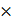# Two kg of a monoatomic gas is at a pressure of 4104 N/m2. The density of the gas is 8kg/m3. What is the order of energy of the gas due to its thermal motion? (a) 103 J (b) 105 J (c) 106 J (d) 104 J

## Question ID - 50284 :- Two kg of a monoatomic gas is at a pressure of 4104 N/m2. The density of the gas is 8kg/m3. What is the order of energy of the gas due to its thermal motion? (a) 103 J (b) 105 J (c) 106 J (d) 104 J

3537

Thermal energy of N molecule

=N=RT

=(nRT)

=PV

=P=4×=1.5×104

Order will 104

Next Question :
 A particle which is experiencing a force, given by=312undergoes a displacement of=4. If the particle had a kinetic energy of 3 J at the beginning of the displacement, what is its kinetic energy at the end of the displacement? (a) 15 J (b) 10 J (c) 12 J (d) 9 J